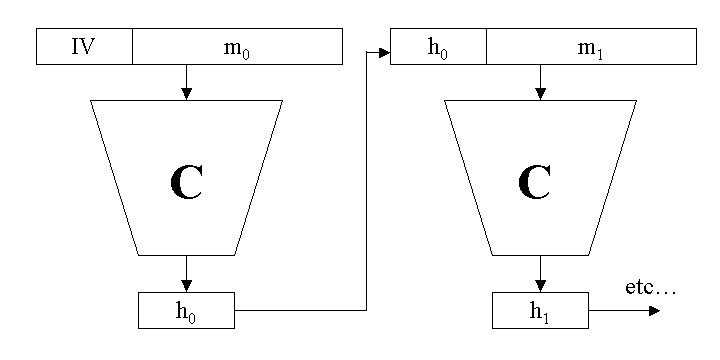# Iterative hashes

Martin McBride, 2017-04-09
Tags cryptography hashing iterative hash
Categories cryptography

Most common hash algorithms are block based, and rely on a compression function C. The compression function has a block size B, and an output size L (which also corresponds to the hash size).

The compression function looks like this:

z = C(x, y)


Here x is a quantity of length B bits, y is a quantity of length L bits, and the result z contains L bits. C is generally quite a complex function for which any small change in x or y creates a large change in z. It is called a compression function simply because it reduces a larger quantity of bits (B+L) to a smaller quantity, L bits (it has nothing to do with ZIP compression, you certainly cannot reverse the function to find a and b from x).

To calculate the hash of a message, the message is divided into n blocks m0 to mn-1, each of size B bits. If necessary the data is padded to form complete blocks (more below). The compression function is applied iteratively:

h<sub>0</sub> = C(m<sub>0</sub>, IV)
...
h<sub>i</sub> = C(m<sub>i</sub>, h<sub>i-1</sub>)
...
h<sub>n-1</sub> = C(m<sub>n-1</sub>, h<sub>n-2</sub>)


The initial value IV is a fixed value (it is algorithm specific) - it is L bits long. The hash value is the final output hn-1.In order to calculate the hash for a stream of bits of arbitrary size, the data must be padded to an exact multiple of the block size. Padding is algorithm specific. The most common scheme if fairly simple – append a single 1 bit, a number of 0 bits, followed by the size of the unpadded message in bits (as a 64 bit integer), such that the padded message is an exact multiple of B bits. This scheme protects against the cases where very similar messages of slightly different length might have the same hash.

Copyright (c) Axlesoft Ltd 2021